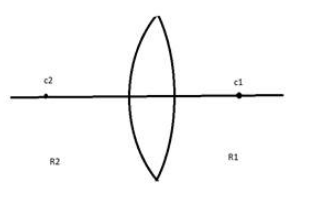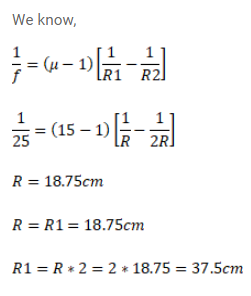# A double convex lens has focal lengthQuestion:

A double convex lens has focal length $25 \mathrm{~cm}$. The radius of curvature of one of the surface is double of the other. Find the radii, if the refractive index of the material of the lens is 1.5.Solution: